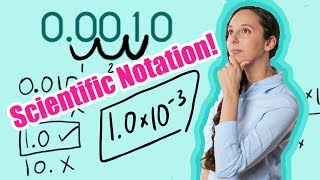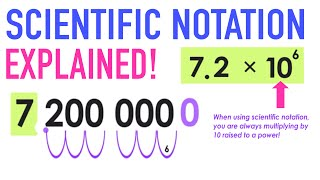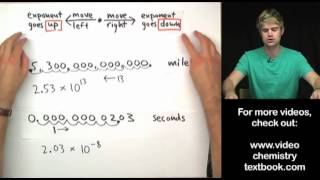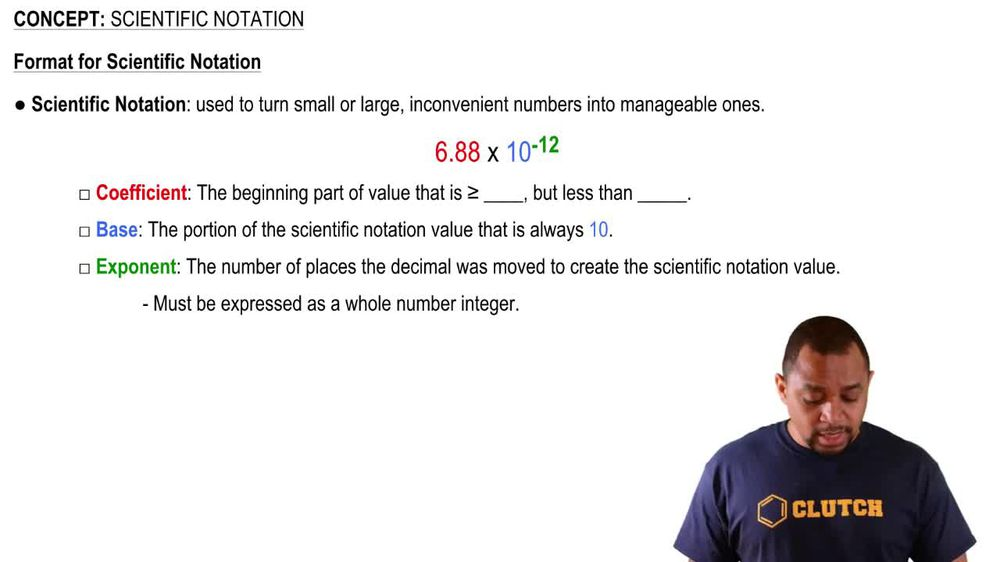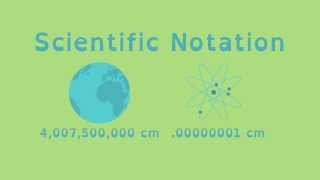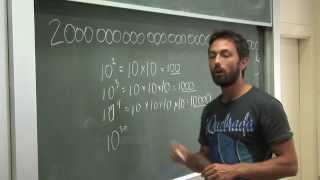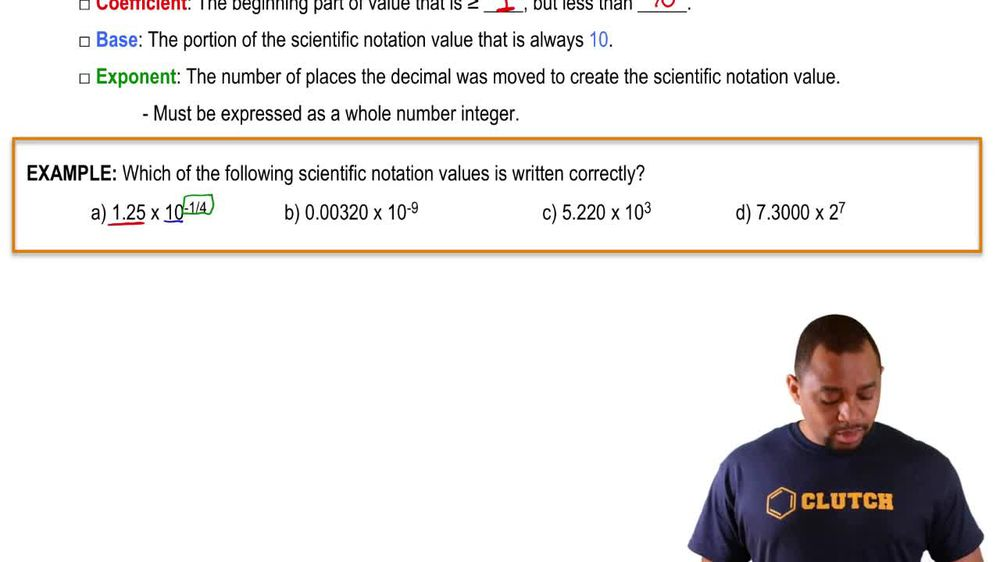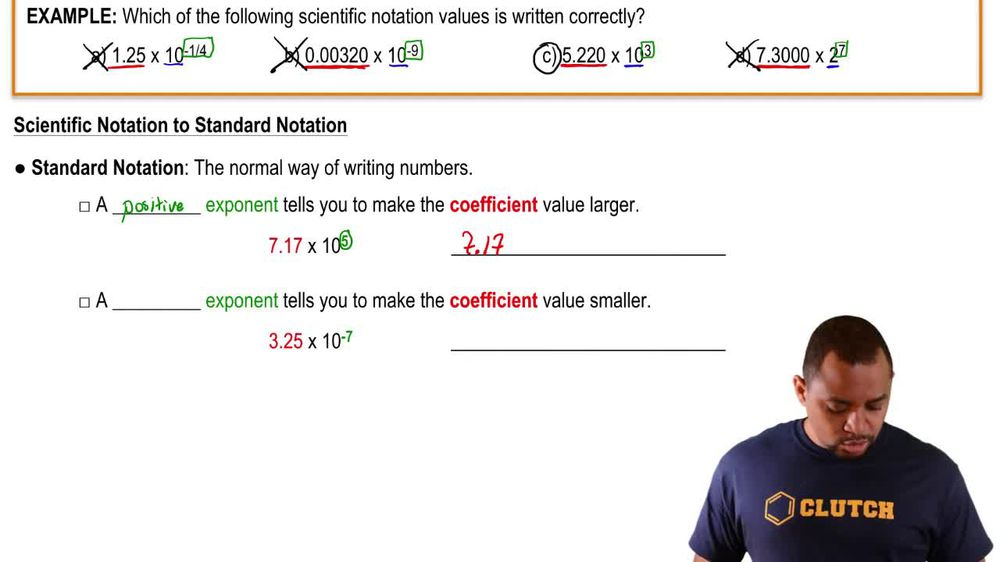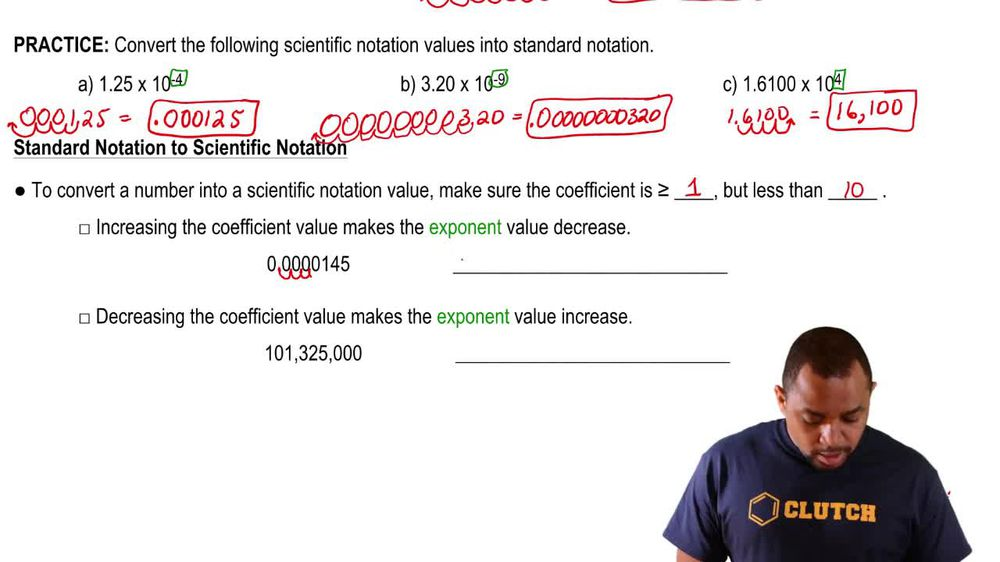Start typing, then use the up and down arrows to select an option from the list.
1. 1. Intro to General Chemistry2. Scientific Notation
Problem

# Use scientific notation to express each quantity with only base units (no prefix multipliers). a. 4.5 ns b. 18 fs c. 128 pm d. 35 mm

Relevant Solution46s
Play a video:
Hi everyone today, we have a question asking us to express the following using base units millimeters. So first we have to remember that one millimeter Equals 10 to the negative 3rd m. And now that we know that we can make an equation, We're gonna start out using our 48 mm And then we're going to multiply that by 10 to the negative 3rd m over one millimeter, And that equals 4.8 Times to the -2 m. And that is our final answer. Thank you for watching. Bye.